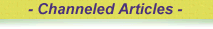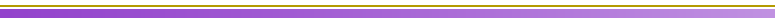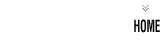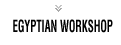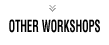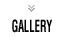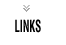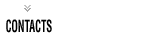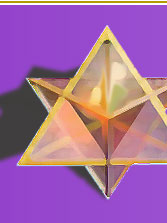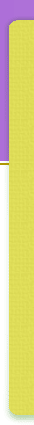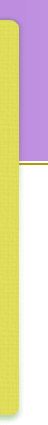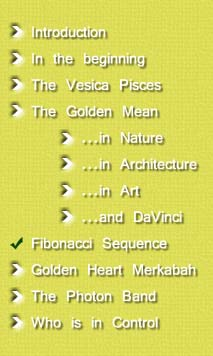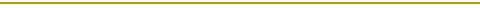Leonardo Fibonacci was born some time before DaVinci. He was a monk in a contemplative order who's job it was to maintain the gardens. As he went about his work in a meditative state he noticed a pattern in the structure of the trees and flowers in the garden. This pattern has become known as the Fibonacci Series. In the table below, look at the two left columns, the Current Term and the Previous Term. We start with a 1 in the current term and take it over into the previous term. We then add these together and place the result in the current term. We then take the number above the 2 and place it in the previous term column, and then add these two together, placing the result in the current term. Keep repeating this process to create the Fibonacci sequence.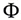= 1.6180339.. (Fibonacci Sequence) Current Term Previous Term Division Ratio 1 1 1 / 1 1.0 2 1 2 / 1 2.0 3 2 3 / 2 1.5 5 3 5 / 3 1.6666 8 5 8 / 5 1.600 13 8 13 / 8 1.625 21 13 21 / 13 1.615384 34 21 34 / 21 1.619048 55 34 55 / 34 1.617647 89 55 89 / 55 1.618182 144 89 144 / 89 1.617978 233 144 233 / 144 1.618056 If we take our attention now to the two columns on the right, we see that these are named Division and Ratio. We now take the numbers generated in the two left-hand columns and transpose them into the two right columns. By dividing the number from the Previous Term column into the number from the Current Term column we arrive with a number in the Ratio column. As you perform this simple calculation down the number sequence you will see that the number generated in the Ratio column gradually aligns with the Fibonacci Sequence. But what does this mean?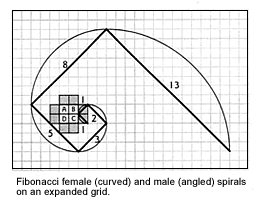Leonardo Fibonacci discovered that the number of petals on a flower, or the number of leaves on a branch always followed the same pattern. The number of leaves or petals would always equal 3,5,8,13,21 etc. What he discovered was that nature itself is built upon the building block of Phi = 1.618. A more common way of showing how the Golden Mean, through the Fibonacci Series, creates the world around us is by creating a spiral using the Fibonacci number sequence. In Drunvalo's book he shows how to generate a Fibonacci spiral using graph paper.We start at the top pf the number sequence and place two squares side by side creating a Golden Mean Rectangle. We then create the next number in the sequence using the squares and make a right-angle turn. We keep making a right-angled turn as we create the Fibonacci sequence. As can be seen this quickly generates a spiral. As Drunvalo shows it the right-angled lines are the male energy of the spiral, and the curved line is the female energy.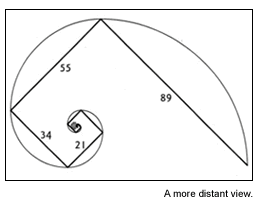An occult truth is hidden here pertaining to ascension. When we chose to come into incarnation we always come in on a spiral. When ascending the planes we always ascend at right-angles. So how does this relate to our world? All natural spirals are created using this number sequence as a building block. The number sequence is based on the Golden Mean value of 1.618 and so proves again that the 'calling card' of God, of Creation, is ever present around us. Here are some examples of this.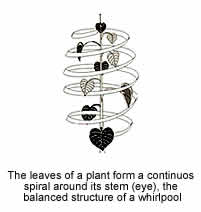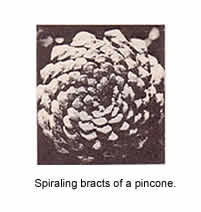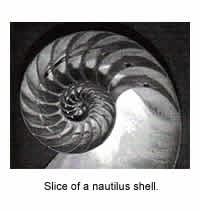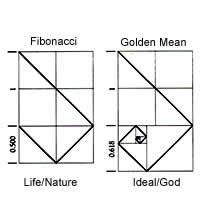The diagram above shows the mismatch between the Fibonacci and nature which ensures the diversity of nature. Drunvalo also talks about a building 1 mile from the Great Pyramid at Giza. In this building is a perfect spherical shaft which sinks into the Earth for 1 mile. In the Emerald Tablets of Thoth it states that this shaft was created at the time of the Great Pyramid, and all other pyramid structures on the Earth. If a you take a Fibonacci Spiral from this spot and spiral it our across the Earth, it passes through every pyramid structure upon the Earth, including the Great Pyramid of Giza. This is because, at the time of the Ascension, a crystal capstone will be lowered onto the top of the Great Pyramid of Giza and a pulse of energy from the Galactic Core will radiate out from this point into the grids of the Earth, impulsing the Ascension of the planet.